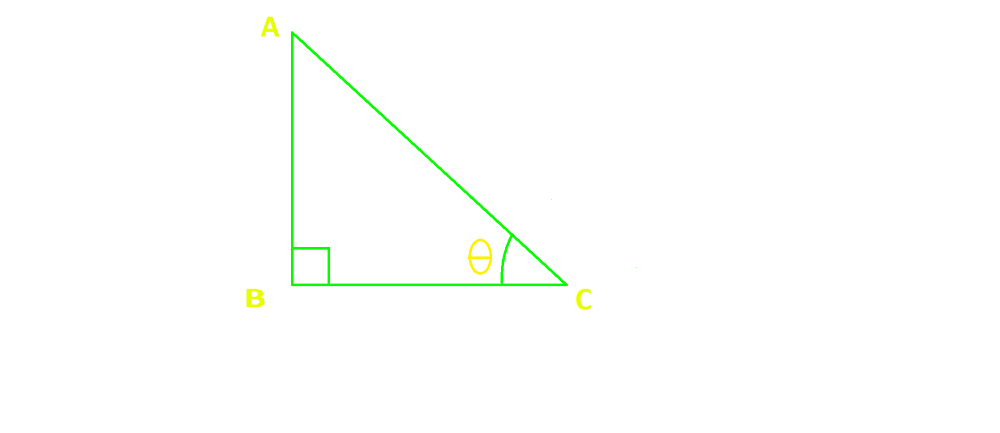# Sin Squared x formula

• Difficulty Level : Basic
• Last Updated : 01 May, 2022

The word Trigonometry is derived from two Greek words: “trigon” meaning is ‘a triangle’ and metron meaning  ‘to measure ‘. Simply, Trigonometry means ‘measurement of triangles’. So, we can say that trigonometry is that branch of mathematics that deals with the measurement of the sides and the angles of a triangle and the problems allied with the angles.

### Sin squared x formula

According to a more general definition, an angle is defined to be ‘the amount of revolution that a line undergoes, while revolving about one of its extremities in passing from a fixed initial position to another position.

1. Sin2θ + Cos2θ = 1

Derivation: Let us considered a triangle ABC,we have, Sin2θ + Cos2θ = 1 ……….(i)

As we know,

Sinθ = Perpendicular/Hypotenuse = AB/AC

Cosθ = Base/Hypotenuse = BC/AC

From eq(i), we get

LHS: Sin2θ + Cos2θ

(AB/AC)2 + (BC/AC)  ……….(ii)

AB2 + BC2/AC2

Using Pythagorean Theorem,  (H2 = P2 + B2)

Here, H = Hypotenuse

P = Perpendicular

B = base

(AC)2 = (AB)2 + (BC)2

From eq (ii)

AC2/AC2 = 1

Hence, LHS = RHS

2. Sin2X = 1 – Cos2X/2

Derivation: We have studied some identies of trigonometric, One of them is

Cos(A + B) = CosACosB – SinASinB

Let us considered both A and B as X,

Now, it became

Cos(X+X) = CosXCosX – SinXSinX

Cos2X = Cos2x – Sin2X   ………..(1)

Earlier we studied, Sin2x + Cos2x = 1

So, from above equation : Cos2x = 1 – Sin2x

Now, equation (1)

Cos2x = 1 – Sin2x – Sin2x

Cos2x = 1 – 2Sin2x

– 2Sin2x = Cos2x – 1

Sin2x = 1 – Cos2x/2

Hence derived.

### Sample problems

Question 1: Solve the equation: 4Cos2x + 6Sin2x = 5

Solution:

The given equation is 4Cos2x + 6Sin2x = 5

As we know Sin2θ + Cos2θ = 1

4(1 – Sin2x) + 6 Sin2x = 5

4 – 4 Sin2x + 6 Sin2x = 5

2Sin2x = 1

Sin2x = 1/2

Sinx = 1/√2

x = sin-11/√2

x = π/4

Question 2: Solve the equation: 2Sin2x + Sin22x = 2

Solution:

The given equation is 2Sin2x + Sin22x = 2

2Sin2x + (2SinxCosx)2 = 2

2Sin2x + 4Sin2xCos2x = 2

We see earlier, Sin2X = 1 – Cos2X/2

2(1 – Cos2x) + 4Sin2xCos2x = 2

2Cos2x(-1 + 2Sin2x) = 0

Case 1: 2Cos2x = 0

Cos2x = 0

Cos x = 0

x =π/2

Case 2: -1 + 2Sin2x = 0

2Sin2x = 1

Sin2x = 1/2

Sinx = 1/√2

x = sin-11/√2

x = π/4

Question 3: Solve the equation: 2Cos2x – 5Sinx + 1 = 0

Solution:

The given equation is 2Cos2x – 5Sinx + 1 = 0

2(1 – Sin2x) – 5Sinx + 1 = 0

-2Sin2x + 2 – 5Sinx + 1 = 0

2Sin2x + 5Sinx – 3 = 0

2Sin2x + 6Sinx – Sinx – 3 = 0

2Sinx(Sinx + 3) – 1(Sinx + 3) = 0

(2Sinx – 1) + (Sinx – 3) = 0

Case 1: 2Sinx – 1 = 0

2Sinx = 1

Sinx = 1/2

x = π/6

Case 2: Sinx – 3 = 0

Sinx = 3

Not Possible.

Question 4: Solve the equation: Cot2α + 3/Sinα + 3 = 0

Solution:

The given equation is Cot2α + 3/Sinα + 3 = 0

Cos2α/Sin2α + 3/Sinx + 3 = 0

Cos2x + 3Sinx+ 3Sin2x = 0

1 – Sin2x + 3Sinx +  3Sin2x = 0

2Sin2x + 3Sinx + 1 = 0

2Sin2x + 2Sinx + Sinx + 1 = 0

2Sinx(Sinx + 1) + 1(Sinx + 1) = 0

Case 1: sinx + 1 = 0

sinx = -1

x = -π/2

Case 2: 2Sinx + 1 = 0

sinx = -1/2

x = -sin-11/2

x = -π/6

Question 5: Solve the equation: Cosx – Sinx = 1/√2

Solution:

The given equation is Cosx – Sinx = 1/√2

Squaring both sides,

(Cosx – Sinx)2 = (1/√2)2

Cos2x + Sin2x – 2SinxCosx = 1/2

(1) – sin2x = 1/2

Sin2x = 1/2

2x = π/6

x = π/12

My Personal Notes arrow_drop_up
Recommended Articles
Page :